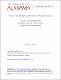## Several types of groupoids induced by two-variable functions2016
Springer
##### Abstract

In this paper, we introduce the concept of several types of groupoids related to semigroups, viz., twisted semigroups for which twisted versions of the associative law hold. Thus, if (X, *) is a groupoid and if phi : X-2 -> X-2 is a function phi (a, b) = (u, v), then (X, *) is a left-twisted semigroup with respect to phi if for all a, b, c is an element of X, a * (b * c) = (u * v) * c. Other types are right-twisted, middle-twisted and their duals, a dual left-twisted semigroup obeying the rule (a * b) * c = u * (v * c) for all a, b, c is an element of X. Besides a number of examples and a discussion of homomorphisms, a class of groupoids of interest is the class of groupoids defined over a field (X,+, .) via a formula x * y = lambda x + mu y, with lambda, mu is an element of X, fixed structure constants. Properties of these groupoids as twisted semigroups are discussed with several results of interest obtained, e.g., that in this setting simultaneous left-twistedness and right-twistedness of (X, *) implies the fact that (X, *) is a semigroup.

##### Keywords
Groupoid, (Twisted) semigroup, Linear groupoid over a field, nth power property, Homomorphism, Multidisciplinary Sciences, Science & Technology - Other Topics
##### Citation
Allen, P., Kim, H., Neggers, J. (2016): Several Types of Groupoids Induced by Two-Variable Functions. SpringerPlus. Volume 5.